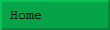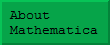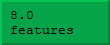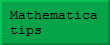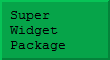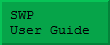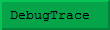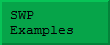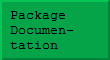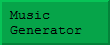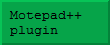Download notebook A substantial number of researchers want to use Mathemnatica rather like a sophisticated calculator. After all, in a fraction of a second it can perform algebraic or calculus operations which would require days or weeks of effort – and it is far more accurate than a human being could ever be. However, you are unlikely to get the best from Mathematica if you regularly start each session with an empty notebook. For example, suppose for your application you wanted to enter r/theta pairs (using degrees, not radians) and for them to automatically convert to x/y vectors using Real numbers. You might enter a definition such as: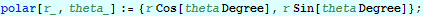This would enable you to enter terms such as polar[4.5,30], and obtain a regular Mathematica list representation of an x/y vector. This is fine, but people often look at a definition such as this and ask if it is really worth entering even such a simple definition (and debugging it!) just to convert a few vectors. Possibly they even start doing it explicitly to save the bother of setting up the function. It is vital to realise that to use Mathematica effectively, you should build up a notebook (effectively your toolbox) of definitions that you enter each time you start Mathematica. This code will gradually grow as you learn more techniques, and it means that Mathematica becomes better and better at solving your particular problems. It is even possible to arrange for code to be executed automatically as Mathematica starts up. This can be extremely useful, but in the early stages it may be better to execute your start-up code explicitly, just so that you remember what it is you are doing.